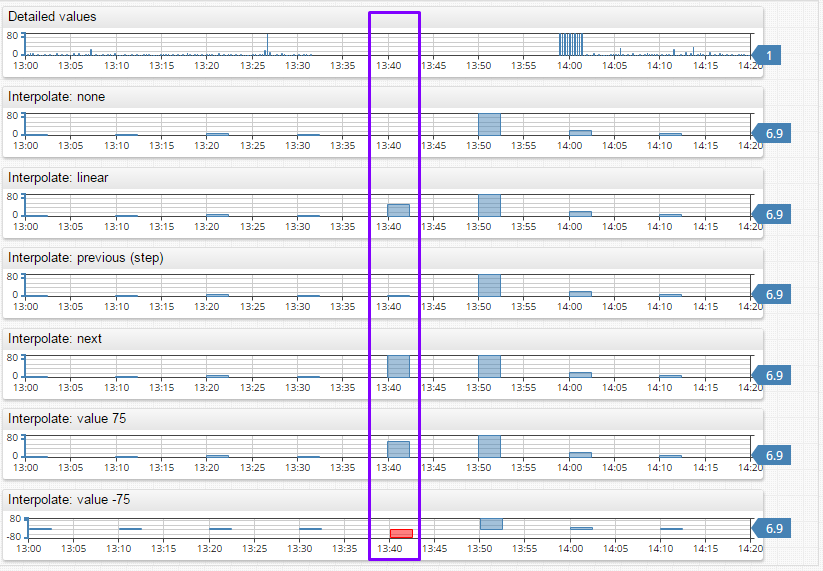# # Aggregate Processor

## # Overview

Aggregate Processor splits an interval into periods and calculates statistics for each period.

The aggregation process is implemented as follows:

1. Load detailed data within the specified `startDate` and `endDate` into each series separately.
`startDate` is inclusive and `endDate` is exclusive.
2. Allocate `time:value` samples to periods based on a period alignment parameter.
If period is not specified in the request, statistics are computed for the entire selection interval.
3. Discard periods whose start time is earlier than `startDate`.
4. Apply statistical function to values in each period and return a modified `time:value` array for each series where `time` is the period start time and `value` is the result of the statistical function.

## # Fields

Name Type Description
`type` string [type or types is Required] Statistical function applied to detailed values in each period, such as `AVG` or `COUNT`.
The field can also be set to `DETAIL` in which case no aggregation is performed and the underlying series is returned unchanged.
`types` array [type or types is Required] Array of statistical functions. Each function in the array produces a separate aggregated series. If one of the functions is set to `DETAIL`, its result contains the underlying series.
`period` object Regular period specified with count and time unit, for example
`{ "count": 1, "unit": "HOUR" }`.
`interpolate` object Fill values in empty periods using an interpolation function such as `PREVIOUS` or `LINEAR`.

### #Threshold Function Fields

Name Type Description
`threshold` object Object containing the minimum and maximum range for `THRESHOLD_*` functions.
`calendar` object Calendar settings for `THRESHOLD_*` functions.
`workingMinutes` object Working minutes settings for `THRESHOLD_*` functions.

### # Period

Period is a repeating time interval used to group detailed values within some timespan to apply a statistical function.

Name Type Description
`unit` string Time unit such as `MINUTE`, `HOUR`, `DAY`.
`count` number Number of time units contained in the period.
`align` string Alignment of the period start/end time.
Allowed values: `CALENDAR`, `START_TIME`, `END_TIME`, `FIRST_VALUE_TIME`.
Default: `CALENDAR`.
`timezone` string Time Zone ID for aligning timestamps in `CALENDAR` mode.
The time zone is inherited from the corresponding setting at the query level and defaults to the database time zone displayed on the Settings > System Information page.

Examples:

• `{ "count": 1, "unit": "HOUR" }`
• `{ "count": 15, "unit": "MINUTE", "align": "END_TIME" }`.

### # Calendar

Name Type Description
`name` string Custom calendar name

Example: `{ "name": "au-nsw-calendar" }`.

### # Threshold

• Either `min` or `max` is required.
Name Type Description
`min` number Minimum threshold.
`max` number Maximum threshold.

Example: `{ "max": 80 }` or `{ "min": 100, "max": 150 }`.

### # Working Minutes

Name Type Description
`start` number [Required] Working date start time, in minutes. If working day starts at 9:30 then `start` can be specified as `570` (`9 * 60 + 30`).
`end` number [Required] Working date end time, in minutes.

## # Examples

• 1-hour Average
``````{
"aggregate" : {
"type": "AVG",
"period": {"count": 1, "unit": "HOUR"}
}
}
``````
• 15-minute Average and Maximum
``````{
"aggregate": {
"types": [ "AVG", "MAX" ],
"period": { "count": 15, "unit": "MINUTE" }
}
}
``````
• Average and Count for the Entire Interval
``````{
"aggregate": {
"types": [ "AVG", "COUNT" ]
}
}
``````

### # Interpolation

By default, if the period does not contain any detailed values, the period is excluded from results.

Configure this behavior with an interpolation function which fills a missing period with data calculated based on previous and next period aggregate values.

Note

Missing period values are interpolated from aggregate values of neighboring periods and not from detailed values.

#### # Interpolation Fields

Name Type Description
`type` string [Required] Interpolation function.
`value` number [Required by `value` function] Constant number returned for empty periods.
`extend` boolean Add missing periods at the beginning and end of the selection interval.
Default: `false`.

Values added by the `extend` setting are determined as follows:

• If the `VALUE {n}` interpolation function is specified, the `extend` option sets empty leading and trailing period values equal to `{n}`.
• Without the `VALUE {n}` function, the `extend` option adds missing periods at the beginning and end of the selection interval using `NEXT` and `PREVIOUS` interpolation functions.

#### # Interpolation Functions

Type Description
`NONE` No interpolation. Periods without detailed values are excluded from results.
`PREVIOUS` Set value for the period based on the previous period value.
`NEXT` Set value for the period based on the next period value.
`LINEAR` Calculate period value using linear interpolation between previous and next period values.
`VALUE` Set value for the period to a specific number.

#### # Examples

PREVIOUS:

``````"aggregate" : {
"type": "AVG",
"period": {"count": 1, "unit": "HOUR"},
"interpolate" : {
"type": "PREVIOUS"
}
}
``````

LINEAR:

``````"aggregate" : {
"type": "AVG",
"period": {"count": 1, "unit": "HOUR"},
"interpolate" : {
"type": "LINEAR"
}
}
``````

VALUE WITH EXTEND:

``````"aggregate" : {
"type": "SUM",
"period": {"count": 1, "unit": "HOUR"},
"interpolate" : {
"type": "VALUE",
"value": 0,
"extend": true
}
}
``````